#### NCERT Solutions for Class 12th: Ch 2 Inverse Trigonometric Functions Miscellaneous Exercise Math

Page No: 51

Miscellaneous Exercise on Chapter 2

Find the value of the following:

Question: 1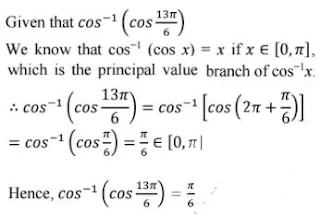Question: 2Prove that

Question: 3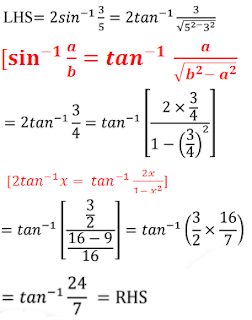Question: 4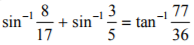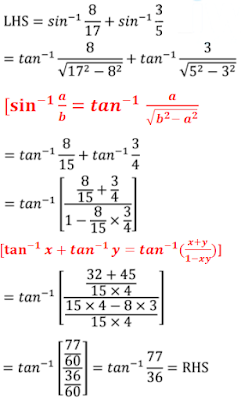Question: 5Question: 6Question: 7Question: 8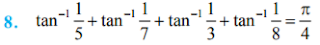Page No. 52

Prove that

Question: 9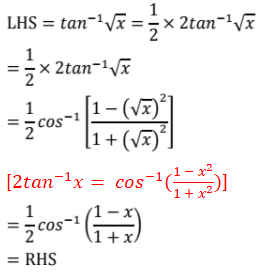Question: 10Question: 11Question: 12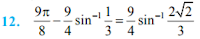Solve the following equations:

Question: 13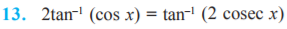Question: 14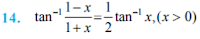Question: 15The correct option is D.

Question: 16
sin–1(1 – x) – 2 sin–1x = π/2, then x is equal to
(A) 0, 1/2
(B) 1, 1/2
(C) 0
(D) 1/2

Given that sin–1(1 − x) − 2sin–1x = π/2
Let x = sin y
∴ sin–1(1 − sin y) − 2y = π/2
⇒ sin–1(1 − sin y) = π/2 + 2y
⇒ 1 − sin y = sin (π/2 + 2y)
⇒ 1 − sin y = cos 2y
⇒ 1 − sin y = 1 − 2sin2y [as cos2y = 1−2sin2y]
⇒ 2sin2y − sin y = 0
⇒ 2x2 − x = 0 [as x = sin y]
⇒ x(2x − 1) = 0
⇒ x = 0 or,  x = 1/2

But x = 1/2 does not satisfy the given equation.
∴ x = 0 is the solution of the given equation.
The correct option is C.

Question: 17
tan–1(x/y) − tan–1(x-y/x+y) is equal to
(A) π/2
(B) π/3
(C) π/4
(D) -3π/4